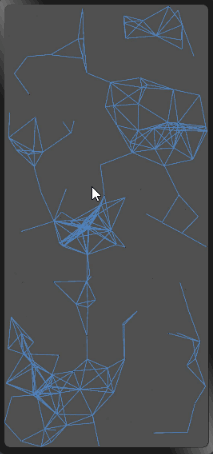# OpenHarmony - 基于ArkUI(JS)实现移动粒子效果背景 原创精华

5收藏

## 效果演示## 实现步骤

### 1. 创建canvas标签

``````<div class="container">
<canvas ref="canvas1" style="width: 100%; height: 100%; background-color: rgb(80, 80, 80);" @touchmove="handleMove"></canvas>
</div>
``````

### 2. 创建粒子

``````export default {
data: {
dots: [], // 存储粒子对象的数组
},
// 创建粒子，并存储到数组中
drawBackground() {
// 创建粒子，并存储到数组中
for (let i=0; i<180; i++) {
// 粒子的位置
let x = Math.random() * 360
let y = Math.random() * 780

// 水平移动的速度，垂直移动的速度
let xa = Math.random() * 0.5
let ya = Math.random() * 0.5
this.dots.push({ x, y, xa, ya, max: 3600 })
}
},
}
``````

### 3. 手指触摸事件

web端的效果是跟随鼠标的移动，移动端没有鼠标，那我们就让粒子向手指移动的地方靠近。用手指触摸事件来模拟鼠标移动事件。

`x`代表手指触摸屏幕的横坐标，`y`代表手指触摸屏幕的纵坐标，`max`代表粒子向手指触摸屏幕位置靠近的最小距离条件。

`handleMove`事件的作用，手指触摸屏幕时更新手指的坐标位置。

``````export default {
data: {
mouse: { // 手指位置
x: null,
y: null,
max: 3200
}
},
handleMove(e) {
this.mouse.x = e.touches.localX
this.mouse.y = e.touches.localY
}
}
``````

### 4. 粒子的运动

``````draw(ctx) {
const self = this;
// 清空整个画布
ctx.clearRect(0, 0, 360, 780);
// 粒子和鼠标的结合，把鼠标数组添加到粒子数组中
const nDots = [this.mouse, ...this.dots];
// 粒子运动
this.dots.forEach(function (dot) {
dot.x += dot.xa;
dot.y += dot.ya;
// 粒子运动的边界（画布），反弹
dot.xa *= (dot.x > 360 || dot.x < 0) ? -1 : 1;
dot.ya *= (dot.y > 780 || dot.y < 0) ? -1 : 1;
// 绘制粒子
ctx.fillRect(dot.x, dot.y, 1, 1);
ctx.fillStyle = "#282828";
// 连线
for (let i=0; i<nDots.length; i++) {
let d = nDots[i];
if(d == dot) {
continue;
}
// 计算粒子的距离
let dx = dot.x - d.x;
let dy = dot.y - d.y;
let dDistance = Math.pow(dx, 2) + Math.pow(dy, 2);
// 连线操作
if (dDistance < d.max) {
// 处理触摸事件
if (d == self.mouse && dDistance > d.max / 2) {
dot.x -= dx * 0.03;
dot.y -= dy * 0.03;
}
// 绘制线条
ctx.beginPath();
ctx.lineWidth = 0.7;
ctx.strokeStyle = 'rgba(80, 130, 189, 0.9)';
// 起始位置
ctx.moveTo(dot.x, dot.y);
// 结束位置
ctx.lineTo(d.x, d.y);
ctx.stroke();
ctx.closePath();
}
}
// 删除比较过的粒子
nDots.splice(nDots.indexOf(dot), 1);
})
},
``````

### 5. 展示背景

``````export default {
onShow() {
this.drawBackground();
},
drawBackground() {
// 创建粒子，并存储到数组中
for (let i=0; i<180; i++) {
// 粒子的位置
let x = Math.random() * 360;
let y = Math.random() * 780;

// 水平移动的速度，垂直移动的速度
let xa = Math.random() * 0.5;
let ya = Math.random() * 0.5;

this.dots.push({ x, y, xa, ya, max: 3600 }); // 两个粒子相吸的最小距离
}
const can = this.\$refs.canvas1;
const ctx = can.getContext('2d');
setInterval(() => {
this.draw(ctx);
}, 1000 / 60);
}
}
``````

## 总结

1. 处理粒子向手指对象运动时，为了让速度不会太快，增加条件`dDistance > d.max / 2`
2. 当粒子运动边界时，需要给粒子一个反方向的速度；
3. `onInit`生命周期中无法拿到`canvas``dom`，需要在`onShow`方法中获取；
4. 粒子运动的速度看起来不太柔和，需要调试下参数。

## 更多原创内容请关注：中软国际 HarmonyOS 技术团队

5

7条回复

/

2022-9-16 10:04:40

2022-9-16 10:36:17
0aaron

2022-9-16 16:23:10

2022-9-16 16:31:31

2022-9-16 16:32:26

2022-9-16 16:35:05

2022-9-16 18:22:30帖子
视频
声望
粉丝
最近发布
社区精华内容